College Physics : Torque

Example Questions

Example Question #11 : Forces

A mechanic is able to exert a maximum torque of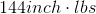using a small wrench. The mechanic adds an attachment to the wrench that doubles the handle length. What is the maximum amount of torque the mechanic can exert with the new wrench?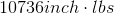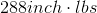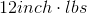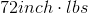Explanation:

The maximum amount of torque is the magnitude of the torque vector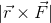, which is maximized when the angle between the applied force and the displacement vector is 90 degrees. Then the torque is given by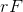, whereis the length of the wrench andis the magnitude of the force applied. Since the mechanic doesn't get any stronger, but the handle length increases, the torque doubles.

Example Question #2 : Torque

An object weighing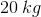sits on a board at a distance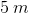away from a fulcrum. At what distance to the right of the fulcrum would an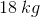object need to be in order to make the board flat?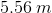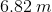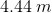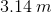Explanation:

For this question, we'll need to take torque into account. The amount of torque caused by the object to the left of the fulcrum needs to be equal to the torque of the object to the right of the fulcrum.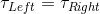Moreover, we can write out the equation for torque.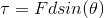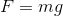Combining these equations, we can set an expression for the torque on the left and the torque on the right.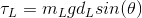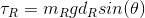Then we can set them equal to each other.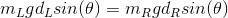Next, we isolate the term for the distance on the right and we cancel out common units.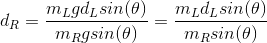Finally, we plug in the values given in the question stem. Because we're told that both objects are sitting flat on the board, we know the force of their weight is perpendicular to the board, which gives a value of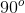.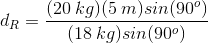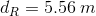All College Physics Resources